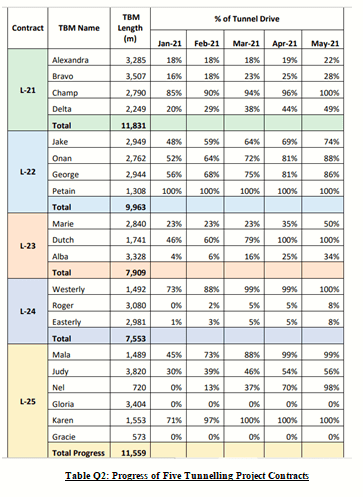# Project Scheduling and Control

The duration and direct cost for each activity in the network of a small construction contract under both normal and crash conditions are given in Table Q1.

Determine the following:

(a) Cost slope for each activity.

(b) Normal cost of the project.

(c) Cost associated with crashing each activity till a stable critical path is obtained.

(d) Provide reasoning for choosing the activity at each stage of (c) above.

(e) Least cost and crash duration.

(f) What conclusion can you draw from the results?

For your computation, use Indirect (overhead) costs of \$125 per day.Question 2

Apply scheduling and control techniques to improve project processes.

The progress of a tunneling project that involves five contracts has been given in Table Q2. Each project uses a few Tunnel Boring Machines (TBM) that drill the ground to make a tunnel. The table shows the Contract name, TBM name, Length that the TBM has to bore, and the progress achieved by each in five calendar months.

Analyze the data and compute the following:

(a) Which machine has made the maximum progress?

(b) Which Contract has made the maximum progress?

(c) Which month has the highest progress – by machine and Contract.

(d) What are the overall progress in percentage (%) and meters?

(e) Which project is expected to finish the last?